# The Many Ways to Analyse Gradient Descent: Part 2

The previous post detailed a bunch of different ways of proving the convergence rate of gradient descent:

${x}_{k+1}={x}_{k}-\alpha {f}^{\prime }\left({x}_{k}\right),$

for strongly convex problems. This post considers the non-strongly convex, but still convex case.

### Rehash of Basic Lemmas

These hold for any $x$ and $y$. $L$ the Lipschitz smoothness constant. These are completely standard, see Nesterov’s book  for proofs. We use the notation ${x}^{\ast }$ for an arbitrary minimizer of $f$.

 $f\left(y\right)\le f\left(x\right)+⟨{f}^{\prime }\left(x\right),y-x⟩+\frac{L}{2}{∥x-y∥}^{2}.$ (1)
 $f\left(y\right)\ge f\left(x\right)+⟨{f}^{\prime }\left(x\right),y-x⟩+\frac{1}{2L}{∥{f}^{\prime }\left(x\right)-{f}^{\prime }\left(y\right)∥}^{2}.$ (2)
 $⟨{f}^{\prime }\left(x\right)-{f}^{\prime }\left(y\right),x-y⟩\ge \frac{1}{L}{∥{f}^{\prime }\left(x\right)-{f}^{\prime }\left(y\right)∥}^{2}.$ (3)

### 1 Proximal Style Convergence Proof

The following argument gives a proof of convergence that is well suited to modiﬁcation for proving the convergence of proximal gradient methods. We start by proving a useful lemma:

Lemma 1. For any ${x}_{k}$ and $y$, when ${x}_{k+1}={x}_{k}-\frac{1}{L}{f}^{\prime }\left({x}_{k}\right)$:

$\frac{2}{L}\left[f\left(y\right)-f\left({x}_{k+1}\right)\right]\ge {∥y-{x}_{k+1}∥}^{2}-{∥{x}_{k}-y∥}^{2}.$

Proof. We start with the Lipschitz upper bound around ${x}_{k}$ of ${x}_{k+1}$:

$f\left({x}_{k+1}\right)\le f\left({x}_{k}\right)+⟨{f}^{\prime }\left({x}_{k}\right),{x}_{k+1}-{x}_{k}⟩+\frac{L}{2}{∥{x}_{k+1}-{x}_{k}∥}^{2}.$

Now we bound $f\left({x}_{k}\right)$ using the negated convexity lower bound of $y$ around $x$ (i.e. $f\left(y\right)\ge f\left({x}_{k}\right)+⟨{f}^{\prime }\left({x}_{k}\right),y-{x}_{k}⟩$):

$f\left({x}_{k+1}\right)\le f\left(y\right)+⟨{f}^{\prime }\left({x}_{k}\right),{x}_{k+1}-{x}_{k}+{x}_{k}-y⟩+\frac{L}{2}{∥{x}_{k+1}-{x}_{k}∥}^{2}.$

Negating, rearranging and multiplying through by $\frac{2}{L}$ gives:

$\frac{2}{L}\left[f\left(y\right)-f\left({x}_{k+1}\right)\right]\ge \frac{2}{L}⟨{f}^{\prime }\left({x}_{k}\right),{x}_{k+1}-y⟩+{∥{x}_{k+1}-{x}_{k}∥}^{2}.$

Now we replace ${f}^{\prime }\left({x}_{k}\right)$ using ${x}_{k+1}={x}_{k}-\frac{1}{L}{f}^{\prime }\left({x}_{k}\right)$:

$\begin{array}{rcll}\frac{2}{L}\left[f\left(y\right)-f\left({x}_{k+1}\right)\right]& \ge & 2⟨y-{x}_{k+1}\phantom{\rule{0.3em}{0ex}},{x}_{k}-{x}_{k+1}⟩-{∥{x}_{k}-{x}_{k+1}∥}^{2}& \text{}\\ & =& 2⟨y-{x}_{k}+{x}_{k}-{x}_{k+1}\phantom{\rule{0.3em}{0ex}},{x}_{k}-{x}_{k+1}⟩-{∥{x}_{k}-{x}_{k+1}∥}^{2}& \text{}\\ & =& 2⟨y-{x}_{k},{x}_{k}-{x}_{k+1}⟩+∥{x}_{k}-{x}_{k+1}∥{.}^{2}& \text{}\end{array}$

Now we complete the square using the quadratic ${∥y-{x}_{k}+{x}_{k}-{x}_{k+1}∥}^{2}={∥y-{x}_{k}∥}^{2}+2⟨y-{x}_{k},{x}_{k}-{x}_{k+1}⟩+{∥{x}_{k}-{x}_{k+1}∥}^{2}$. So we have:

$\begin{array}{rcll}\frac{2}{L}\left[f\left(y\right)-f\left({x}_{k+1}\right)\right]& \ge & ∥y-{x}_{k}+{x}_{k}-{x}_{k+1}∥-{∥y-{x}_{k}∥}^{2}& \text{}\\ & =& ∥y-{x}_{k+1}∥-{∥y-{x}_{k}∥}^{2}.& \text{}\end{array}$

Using this lemma, the proof is quite simple. We apply it with $y={x}^{\ast }$:

${∥{x}_{k+1}-{x}^{\ast }∥}^{2}-{∥{x}_{k}-{x}^{\ast }∥}^{2}\le -\frac{2}{L}\left[f\left({x}_{k+1}\right)-f\left({x}^{\ast }\right)\right].$

Now we sum this between $0$ and $k-1$. The left hand side telescopes:

${∥{x}_{k}-{x}^{\ast }∥}^{2}-{∥{x}_{0}-{x}^{\ast }∥}^{2}\le -\frac{2}{L}\sum _{r=0}^{k-1}\left[f\left({x}_{r+1}\right)-f\left({x}^{\ast }\right)\right].$

Now we use the fact that gradient descent is a descent method, which implies that $f\left({x}_{k}\right)\le f\left({x}_{r+1}\right)$ for all $r\le k-1$. So:

${∥{x}_{k}-{x}^{\ast }∥}^{2}-{∥{x}_{0}-{x}^{\ast }∥}^{2}\le -\frac{2k}{L}\left[f\left({x}_{k}\right)-f\left({x}^{\ast }\right)\right].$

Now we just drop the ${∥{x}_{k}-{x}^{\ast }∥}^{2}$ term since it is positive and small. Leaving:

$f\left({x}_{k}\right)-f\left({x}^{\ast }\right)\le \frac{L}{2k}{∥{x}_{0}-{x}^{\ast }∥}^{2}.$

As far as I know, this proof is fairly modern . Notice that unlike the strongly convex case, the quantity we are bounding $\left(f\left({x}_{k}\right)-f\left({x}^{\ast }\right)\right)$ does not appear on both sides of the bound. Unfortunately, without strong convexity there is necessarily a looseness to the bounds, and this takes the form of bounding function value by distance to solution, with a large wiggle-factor. One thing that is perhaps a little confusing is the use of distance to solution $x-{x}^{\ast }$, when it is not unique, as there are potentially multiple minimizers for non-strongly convex problems. The bound in fact holds for any chosen minimizer ${x}^{\ast }$. I found this to be a little confusing at ﬁrst.

### 2 Older Style Proof

This proof is from Nesterov . I’m not sure of the original source for it.

We start with the function value descent equation, using $w:=\left[\alpha \left(1-\frac{1}{2}\alpha L\right)\right]:$

$f\left({x}_{k+1}\right)\le f\left({x}_{k}\right)-w{∥{f}^{\prime }\left({x}_{k}\right)∥}^{2}.$

We introduce the simpliﬁed notation ${\Delta }_{k}=f\left({x}_{k}\right)-f\left({x}^{\ast }\right)$ so that we have

 ${\Delta }_{k+1}\le {\Delta }_{k}-w{∥{f}^{\prime }\left({x}_{k}\right)∥}^{2}.$ (4)

Now using the convexity lower bound around ${x}_{k}$ evaluated at ${x}^{\ast }$, namely:

${\Delta }_{k}\le ⟨{f}^{\prime }\left({x}_{k}\right),{x}_{k}-{x}^{\ast }⟩,$

and applying Cauchy-Schwarz (note the spelling! there is no “t” in Schwarz) to it:

$\begin{array}{rcll}{\Delta }_{k}& \le & ∥{x}_{k}-{x}^{\ast }∥∥{f}^{\prime }\left({x}_{k}\right)∥& \text{}\\ & \le & ∥{x}_{0}-{x}^{\ast }∥∥{f}^{\prime }\left({x}_{k}\right)∥.& \text{}\end{array}$

The last line is because gradient descent method descends in iterate distance each step. We now introduce the additional notation ${r}_{0}=∥{x}_{0}-{x}^{\ast }∥$. Using this notation and rearranging gives:

$-∥{f}^{\prime }\left({x}_{k}\right)∥\le -{\Delta }_{k}∕{r}_{0}.$

We plug this into the function descent equation (Eq 4) above to get:

${\Delta }_{k+1}\le {\Delta }_{k}-\frac{w}{{r}_{0}^{2}}{\Delta }_{k}^{2}.$

We now divide this through by ${\Delta }_{k+1}$:

$1\le \frac{{\Delta }_{k}}{{\Delta }_{k+1}}-\frac{w}{{r}_{0}^{2}}\frac{{\Delta }_{k}^{2}}{{\Delta }_{k+1}}$

Then divide through by ${\Delta }_{k}$ also:

$\frac{1}{{\Delta }_{k}}\le \frac{1}{{\Delta }_{k+1}}-\frac{w}{{r}_{0}^{2}}\frac{{\Delta }_{k}}{{\Delta }_{k+1}}.$

Now we use the fact that gradient descent is a descent method again, which implies that $\frac{{\Delta }_{k}}{{\Delta }_{k+1}}\le 1,$ so:

$\frac{1}{{\Delta }_{k}}\le \frac{1}{{\Delta }_{k+1}}-\frac{w}{{r}_{0}^{2}}.$

$\therefore \frac{1}{{\Delta }_{k+1}}\ge \frac{1}{{\Delta }_{k}}+\frac{w}{{r}_{0}^{2}}.$

We then chain this inequality for each $k$:

$\frac{1}{{\Delta }_{k+1}}\ge \frac{1}{{\Delta }_{k}}+\frac{w}{{r}_{0}^{2}}\ge \frac{1}{{\Delta }_{k-1}}+2\frac{w}{{r}_{0}^{2}}\ge \cdots \ge \frac{1}{{\Delta }_{0}}+\frac{w}{{r}_{0}^{2}}\left(k+1\right)$

$\therefore \frac{1}{{\Delta }_{k+1}}\ge \frac{1}{{\Delta }_{0}}+\frac{w}{{r}_{0}^{2}}\left(k+1\right).$

To get the ﬁnal convergence rate we invert both sides:

$f\left({x}_{k}\right)-f\left({x}^{\ast }\right)\le \frac{\left[f\left({x}_{0}\right)-f\left({x}^{\ast }\right)\right]{∥{x}_{0}-{x}^{\ast }∥}^{2}}{{∥{x}_{0}-{x}^{\ast }∥}^{2}+w\left[f\left({x}_{0}\right)-f\left({x}^{\ast }\right)\right]k}.$

This is quite a complex expression. To simplify even further, we can get rid of the $f\left({x}_{0}\right)-f\left({x}^{\ast }\right)$ terms on the right hand side using the Lipschitz upper bound about ${x}^{\ast }$:

$f\left({x}_{0}\right)-f\left({x}^{\ast }\right)\le \frac{L}{2}{∥x-{x}^{\ast }∥}^{2}.$

Plugging in the step size $\alpha =\frac{1}{L}$ gives $w=\frac{1}{2L}$, yielding the following simpler convergence rate:

$f\left({x}_{k}\right)-f\left({x}^{\ast }\right)\le \frac{2L{∥{x}_{0}-{x}^{\ast }∥}^{2}}{k+4}.$

Compared to the rate from the previous proof, $f\left({x}_{k}\right)-f\left({x}^{\ast }\right)\le \frac{L}{2k}{∥{x}^{0}-{x}^{\ast }∥}^{2}$, this is slightly better at $k=1$, and worse thereafter.

I don’t like this proof. It’s feels like a random sequence of steps when you ﬁrst look at it. The way the proof uses inverse quantities like $\frac{1}{{\Delta }_{k}}$ is also confusing. The key equation is really the direct bound on $\Delta$:

${\Delta }_{k+1}\le {\Delta }_{k}-\frac{w}{{r}_{0}^{2}}{\Delta }_{k}^{2}.$

Often this is the kind of equation you encounter when proving the properties of dual methods for example. Equations of this kind can be encountered when applying proximal methods to non-diﬀerentiable functions also. It is also quite a clear statement about what is going on in terms of per-step convergence, a property that is less clear in the previous proof.

When we don’t even have convexity, just Lipschitz smoothness, we can still prove something about convergence of the gradient norm. The Lipschitz upper bound holds without the requirement of convexity:

$f\left(y\right)\le f\left(x\right)+⟨{f}^{\prime }\left(x\right),y-x⟩+\frac{L}{2}{∥x-y∥}^{2}.$

Recall that from minimizing this bound with respect to $y$ we can prove the equation:

$f\left({x}_{k}\right)-f\left({x}_{k+1}\right)\ge \frac{1}{2L}{∥{f}^{\prime }\left({x}_{k}\right)∥}^{2}.$

Examine this equation carefully. We have a bound on each gradient encountered during the optimization in terms of the diﬀerence in function values between steps. The sequence of function values is bounded below, so in fact we have a hard bound on the sum of the encountered gradient norms. Eﬀectively, we chain (telescope) the above inequality over steps:

$f\left({x}_{k-1}\right)-f\left({x}_{k}\right)+f\left({x}_{k}\right)-f\left({x}_{k+1}\right)\ge \frac{1}{2L}{∥{f}^{\prime }\left({x}_{k}\right)∥}^{2}+\frac{1}{2L}{∥{f}^{\prime }\left({x}_{k-1}\right)∥}^{2}.$

$...$

$f\left({x}_{0}\right)-f\left({x}_{k+1}\right)\ge \frac{1}{2L}\sum _{i}^{k}{∥{f}^{\prime }\left({x}_{i}\right)∥}^{2}.$

Now since $f\left({x}_{k+1}\right)\ge f\left({x}^{\ast }\right)$:

$\sum _{i}^{k}{∥{f}^{\prime }\left({x}_{i}\right)∥}^{2}\le 2L\left(f\left({x}_{0}\right)-f\left({x}^{\ast }\right)\right).$

Now to make this bound a little more concrete, we can put it in terms of the gradient ${g}_{k}$ with the smallest norm seen during the minimization ($∥{g}_{k}∥\le ∥{g}_{i}∥$ for all $i$), so that ${\sum }_{i}^{k}{∥{f}^{\prime }\left({x}_{i}\right)∥}^{2}\ge k{∥{g}_{k}∥}^{2}$, so:

${∥{g}_{k}∥}^{2}\le \frac{2L}{k}\left(f\left({x}_{0}\right)-f\left({x}^{\ast }\right)\right).$

Notice that the core technique used in this proof is the same as the last 2 proofs. We have a single step inequality bounding one of the quantities we care about. By summing that inequality over each step of minimization, one side of the inequality telescopes. We get an equality saying the the sum of the $k$ versions of that quantity (one from each step) is less then some ﬁxed constant independent of $k$, for any $k$. The convergence rate is thus of the form $1∕k$, because the summation of the $k$ quantities ﬁts in a ﬁxed bound.

Almost any proof for an optimization method that applies in the non-convex case uses a similar proof technique. There is just not that many assumptions to work with, so the options are limited.

### References

   Amir Beck and Marc Teboulle. Gradient-based algorithms with applications to signal recovery problems. Convex Optimization in Signal Processing and Communications, 2009.

   Yu. Nesterov. Introductory Lectures On Convex Programming. Springer, 1998.

# The Many Ways to Analyse Gradient Descent

Consider the classical gradient descent method:
${x}_{k+1}={x}_{k}-\alpha {f}^{\prime }\left({x}_{k}\right).$

It’s a thing of beauty isn’t it? While it’s not used directly in practice any more, the proof techniques used in its analysis are the building blocks behind the theory of more advanced optimization methods. I know of 8 diﬀerent ways of proving its convergence rate. Each of the proof techniques are interesting in their own right, but most books on convex optimization give just a single proof of convergence, then move onto greater things. But to do research in modern convex optimization you should know them all.

The purpose of this series of posts is to detail each of these proof techniques and what applications they have to more advanced methods. This post will cover the proofs under strong convexity assumptions, and the next post will cover the non-strongly convex case. Unlike most proofs in the literature, we will go into detail of every step, so that these proofs can be used as a reference (don’t cite this post directly though, cite the original source preferably, or the technical notes version). If you are aware of any methods I’ve not covered, please leave a comment with a reference so I can update this post.

For most of the proofs we end with a statement like ${A}_{k+1}\le \left(1-\gamma \right){A}_{k}$, where ${A}_{k}$ is some quantity of interest, like distance to solution or function value sub-optimality. A full proof requires chaining these inequalities for each $k$, giving something of the form ${A}_{k}\le {\left(1-\gamma \right)}^{k}{A}_{0}$. We leave this step as a given.

### Basic lemmas

These hold for any $x$ and $y$. Here $\mu$ is the strong convexity constant and $L$ the Lipschitz smoothness constant. These are completely standard, see Nesterov’s book  for proofs. We use the notation ${x}^{\ast }$ for the unique minimizer of $f$ (for strongly convex problems).

 $f\left(y\right)\le f\left(x\right)+⟨{f}^{\prime }\left(x\right),y-x⟩+\frac{L}{2}{∥x-y∥}^{2}.$ (1)
 $f\left(y\right)\ge f\left(x\right)+⟨{f}^{\prime }\left(x\right),y-x⟩+\frac{\mu }{2}{∥x-y∥}^{2}.$ (2)
 $f\left(y\right)\ge f\left(x\right)+⟨{f}^{\prime }\left(x\right),y-x⟩+\frac{1}{2L}{∥{f}^{\prime }\left(x\right)-{f}^{\prime }\left(y\right)∥}^{2}.$ (3)
 $f\left(y\right)\le f\left(x\right)+⟨{f}^{\prime }\left(x\right),y-x⟩+\frac{1}{2\mu }{∥{f}^{\prime }\left(x\right)-{f}^{\prime }\left(y\right)∥}^{2}.$ (4)
 $⟨{f}^{\prime }\left(x\right)-{f}^{\prime }\left(y\right),x-y⟩\ge \frac{1}{L}{∥{f}^{\prime }\left(x\right)-{f}^{\prime }\left(y\right)∥}^{2}.$ (5)
 $⟨{f}^{\prime }\left(x\right)-{f}^{\prime }\left(y\right),x-y⟩\ge \mu {∥x-y∥}^{2}.$ (6)

### 1 Function Value Descent

There is a very simple proof involving just the function values. We start by showing that the function value descent is controlled by the gradient norm:

Lemma 1. For any given $\alpha$, the change in function value between steps can be bounded as follows:

$f\left({x}_{k}\right)-f\left({x}_{k+1}\right)\ge \alpha \left(1-\frac{1}{2}\alpha L\right){∥{f}^{\prime }\left({x}_{k}\right)∥}^{2},$

in particular, if $\alpha =\frac{1}{L}$ we have $f\left({x}_{k}\right)-f\left({x}_{k+1}\right)\ge \frac{1}{2L}{∥{f}^{\prime }\left({x}_{k}\right)∥}^{2}$.

Proof. We start with (1), the Lipschitz upper bound about ${x}_{k}$:

$f\left({x}_{k+1}\right)\le f\left({x}_{k}\right)+⟨{f}^{\prime }\left({x}_{k}\right),{x}_{k+1}-{x}_{k}⟩+\frac{L}{2}{∥{x}_{k+1}-{x}_{k}∥}^{2}.$

Now we plug in the step equation ${x}_{k+1}-{x}_{k}=-\alpha {f}^{\prime }\left({x}_{k}\right):$

$f\left({x}_{k+1}\right)\le f\left({x}_{k}\right)-\alpha {∥{f}^{\prime }\left({x}_{k}\right)∥}^{2}+{\alpha }^{2}\frac{L}{2}{∥{f}^{\prime }\left({x}_{k}\right)∥}^{2},$

Negating and rearranging gives:

$\therefore f\left({x}_{k}\right)-f\left({x}_{k+1}\right)\ge \alpha \left(1-\frac{1}{2}\alpha L\right){∥{f}^{\prime }\left({x}_{k}\right)∥}^{2}.$

Now since we are considering strongly convex problems, we actually have found a bound on the gradient norm in terms of function value. We apply (4): $f\left(y\right)\le f\left(x\right)+⟨{f}^{\prime }\left(x\right),y-x⟩+\frac{1}{2\mu }{∥{f}^{\prime }\left(x\right)-{f}^{\prime }\left(y\right)∥}^{2}$ using $x={x}^{\ast }$, $y={x}_{k}$:

$f\left({x}_{k}\right)\le f\left({x}^{\ast }\right)+\frac{1}{2\mu }{∥{f}^{\prime }\left({x}_{k}\right)∥}^{2},$

$\therefore ∥{f}^{\prime }\left({x}_{k}\right)∥\ge 2\mu \left(f\left({x}_{k}\right)-f\left({x}^{\ast }\right)\right).$

So combining these two results:

$f\left({x}_{k}\right)-f\left({x}_{k+1}\right)\ge \frac{1}{2L}{∥{f}^{\prime }\left({x}_{k}\right)∥}^{2}\ge \frac{\mu }{L}\left(f\left({x}_{k}\right)-f\left({x}^{\ast }\right)\right).$

We then negate, add & subtract $f\left({x}^{\ast }\right)$, then rearrange:

$f\left({x}_{k+1}\right)-f\left({x}_{k}\right)\le -\frac{\mu }{L}\left(f\left({x}_{k}\right)-f\left({x}^{\ast }\right)\right),$

$\therefore f\left({x}_{k+1}\right)-f\left({x}^{\ast }\right)-f\left({x}_{k}\right)+f\left({x}^{\ast }\right)\le -\frac{\mu }{L}\left(f\left({x}_{k}\right)-f\left({x}^{\ast }\right)\right),$

$\therefore f\left({x}_{k+1}\right)-f\left({x}^{\ast }\right)\le \left(1-\frac{\mu }{L}\right)\left(f\left({x}_{k}\right)-f\left({x}^{\ast }\right)\right).$

Note that this function value style proof requires the step size $\alpha =\frac{1}{L}$ or smaller, instead of $\alpha =\frac{2}{\mu +L}$, which we shall see gives the fastest convergence when using some of the other proof techniques below.

This proof (when $\alpha =\frac{1}{L}$ is used) treats gradient descent as an upper bound minimization scheme. Such methods, sometimes known under the Majorization-Minimization nomenclature , are quite widespread in optimization. They can be applied to non-convex problems even, although the convergence rates in that case are necessarily weak. Likewise this proof gives the weakest convergence rate of the proof techniques presented in this post, but it is perhaps the simplest. Upper bound minimization techniques have recently seen interesting applications in 2nd order optimization, in the form of Nesterov’s cubicly regularized Newton’s method . For stochastic optimization, the MISO method is also a upper bound minimization scheme . For non-smooth problems, an interesting application of the MM approach is in minimizing convex problems with non-convex regularizers of the form $\lambda log\left(\left|x\right|+1\right)$, in the form of reweighted L1 regularization .

### 2 Iterate Descent

There is also a simple proof involving just the distance of the iterates ${x}_{k}$ to the solution. Using the deﬁnition of the step ${x}_{k+1}-{x}_{k}=-\alpha {f}^{\prime }\left({x}_{k}\right)$:

$\begin{array}{rcll}{∥{x}_{k+1}-{x}^{\ast }∥}^{2}& =& {∥{x}_{k}-\alpha {f}^{\prime }\left({x}_{k}\right)-{x}^{\ast }∥}^{2}& \text{}\\ & =& {∥{x}_{k}-{x}^{\ast }∥}^{2}-2\alpha ⟨{f}^{\prime }\left({x}_{k}\right),{x}_{k}-{x}^{\ast }⟩+{\alpha }^{2}{∥{f}^{\prime }\left({x}_{k}\right)∥}^{2}.& \text{}\end{array}$

We now apply both the inner product bounds (5) $⟨{f}^{\prime }\left(x\right)-{f}^{\prime }\left(y\right),x-y⟩\ge \frac{1}{L}{∥{f}^{\prime }\left(x\right)-{f}^{\prime }\left(y\right)∥}^{2}$and (6) $⟨{f}^{\prime }\left(x\right)-{f}^{\prime }\left(y\right),x-y⟩\ge \mu {∥x-y∥}^{2}$ , in the following negated forms, using ${f}^{\prime }\left({x}^{\ast }\right)=0$:

$-⟨{f}^{\prime }\left({x}_{k}\right),{x}_{k}-{x}^{\ast }⟩\le -\frac{1}{L}{∥{f}^{\prime }\left({x}_{k}\right)∥}^{2},$

$-⟨{f}^{\prime }\left({x}_{k}\right),{x}_{k}-{x}^{\ast }⟩\le -\mu {∥{x}_{k}-{x}^{\ast }∥}^{2}.$

The inner product term has a weight $2\alpha$, and we apply each of these with weight $\alpha$, giving:

${∥{x}_{k+1}-{x}^{\ast }∥}^{2}\le \left(1-\alpha \mu \right){∥{x}_{k}-{x}^{\ast }∥}^{2}+\alpha \left(\alpha -\frac{1}{L}\right){∥{f}^{\prime }\left({x}_{k}\right)∥}^{2}.$

Now if we take $\alpha =\frac{1}{L},$ then the last term cancels and we have:

${∥{x}_{k+1}-{x}^{\ast }∥}^{2}\le \left(1-\frac{\mu }{L}\right){∥{x}_{k}-{x}^{\ast }∥}^{2}.$

This proof is not as tight as possible. Instead of splitting the inner product term and applying both bounds (5) and (6), we can apply the following stronger combined bound from Nesterov’s Book :

 $⟨{f}^{\prime }\left(x\right)-{f}^{\prime }\left(y\right),x-y⟩\ge \frac{\mu L}{\mu +L}{∥x-y∥}^{2}+\frac{1}{\mu +L}{∥{f}^{\prime }\left(x\right)-{f}^{\prime }\left(y\right)∥}^{2}.$ (7)

Doing so yields:

${∥{x}_{k+1}-{x}^{\ast }∥}^{2}\le \left(1-\frac{2\alpha \mu L}{\mu +L}\right){∥{x}_{k}-{x}^{\ast }∥}^{2}+\alpha \left(\alpha -\frac{2}{\mu +L}\right){∥{f}^{\prime }\left({x}_{k}\right)∥}^{2}.$

Now clearly to cancel out the gradient norm term we can take $\alpha =\frac{2}{\mu +L}$, which yields the convergence rate:

$\begin{array}{rcll}{∥{x}_{k+1}-{x}^{\ast }∥}^{2}& \le & \left(1-\frac{4\mu L}{{\left(\mu +L\right)}^{2}}\right){∥{x}_{k}-{x}^{\ast }∥}^{2}& \text{}\\ & \approx & \left(1-\frac{4\mu }{L}\right){∥{x}_{k}-{x}^{\ast }∥}^{2}.& \text{}\end{array}$

This proof technique is the building block of the standard stochastic gradient descent (SGD) proof. The above proof is mostly based on Nesterov’s book, I’m not sure what the original citation is. It has a nice geometric interpretation, as the bound on the inner product term $⟨{f}^{\prime }\left({x}_{k}\right),{x}_{k}-{x}^{\ast }⟩$ can easily be illustrated in 2 dimensions, say on a white-board. It’s eﬀectively a statement on the angles that gradients in convex problems can take. To get the strongest bound using this technique, the complex bound in Equation 7 has to be used. That stronger bound is not really straight-forward, and perhaps too technical (in my opinion) to use in a textbook proof of the convergence rate.

### 3 Using the Second Fundamental Theorem of Calculus

Recall the second fundamental theorem of calculus:

$f\left(y\right)=f\left(x\right)+{\int }_{x}^{y}{f}^{\prime }\left(z\right)dz.$

This can be applied along intervals in higher dimensions. The case we care about is applying it to the ﬁrst derivatives of $f$, giving an integral involving the Hessian:

${f}^{\prime }\left(y\right)={f}^{\prime }\left(x\right)+{\int }_{0}^{1}⟨{f}^{\prime \prime }\left(x+\tau \left(y-x\right)\right)\phantom{\rule{0.3em}{0ex}},\phantom{\rule{0.3em}{0ex}}y-x⟩d\tau .$

We abuse the angle bracket notation here to apply to matrix-vector products as well as the usual dot-product. Using this result gives an interesting proof of convergence of gradient descent that doesn’t rely on the usual convexity lemmas. This proof bounds the distance to solution, just like the previous proof.

Lemma 2. For any positive $t$:

$∥x-y+t\left({f}^{\prime }\left(y\right)-{f}^{\prime }\left(x\right)\right)∥\le max\left\{\left|1-tL\right|,\left|1-t\mu \right|\right\}∥x-y∥.$

Proof. We start by applying the second fundamental theorem of calculus in the above form:

$\begin{array}{rcll}∥x-y+t\left({f}^{\prime }\left(y\right)-{f}^{\prime }\left(x\right)\right)∥& =& ∥x-y+t{\int }_{0}^{1}⟨{f}^{\prime \prime }\left(x+\tau \left(y-x\right)\right)\phantom{\rule{0.3em}{0ex}},\phantom{\rule{0.3em}{0ex}}y-x⟩d\tau ∥& \text{}\\ & =& ∥{\int }_{0}^{1}⟨t{f}^{\prime \prime }\left(x+\tau \left(y-x\right)\right)-I\phantom{\rule{0.3em}{0ex}},\phantom{\rule{0.3em}{0ex}}y-x⟩d\tau ∥& \text{}\\ & \le & {\int }_{0}^{1}∥⟨t{f}^{\prime \prime }\left(x+\tau \left(y-x\right)\right)-I\phantom{\rule{0.3em}{0ex}},\phantom{\rule{0.3em}{0ex}}y-x⟩∥d\tau & \text{}\\ & \le & {\int }_{0}^{1}∥t{f}^{\prime \prime }\left(x+\tau \left(y-x\right)\right)-I∥∥x-y∥d\tau & \text{}\\ & \le & \underset{z}{max}∥t{f}^{\prime \prime }\left(z\right)-I∥∥x-y∥.& \text{}\end{array}$

Now we examine the eigenvalues of ${f}^{\prime \prime }\left(z\right)$. the minimum one is at least $\mu$ and the maximum at most $L$. An examination of the possible range of the eigenvalues of $\left(t{f}^{\prime \prime }\left(z\right)-I\right)$ gives $max\left\{\left|1-tL\right|,\left|1-t\mu \right|\right\}$. □

Using this lemma gives a simple proof along the lines of the iterate descent proof.

First, note that $∥{x}_{k+1}-{x}^{\ast }∥$ is in the right form for direct application of this lemma after substituting in the step equation:

$\begin{array}{rcll}∥{x}_{k+1}-{x}^{\ast }∥& =& ∥{x}_{k}-{x}^{\ast }+\alpha \left({f}^{\prime }\left({x}_{k}\right)-{f}^{\prime }\left({x}^{\ast }\right)\right)∥& \text{}\\ & \le & max\left\{\left|1-\alpha L\right|,\left|1-\alpha \mu \right|\right\}∥{x}_{k}-{x}^{\ast }∥.& \text{}\end{array}$

Note we introduced ${f}^{\prime }\left({x}^{\ast }\right)$ for “free”, as it’s of course equal to zero. The next step is optimize this bound in terms of $\alpha$. Note that $L$ is always larger than $\mu$, so we take the $\left|1-\alpha L\right|$ absolute value as negative, and the other positive, and match their magnitudes:

$-1+\alpha L=1-\alpha \mu ,$

$\therefore \alpha \left(L+\mu \right)=2,$

$\therefore \alpha =\frac{2}{L+\mu }.$

Which gives the convergence rate:

$∥{x}_{k+1}-{x}^{\ast }∥\le \left(\frac{L-\mu }{L+\mu }\right)∥{x}_{k}-{x}^{\ast }∥.$

Note that this rate is in terms of the distance to solution directly, rather than its square like in the previous proof. Converting to squared norm gives the same rate as before.

This proof technique has a linear-algebra feel to it, and is perhaps most comfortable to people with that background. The absolute values make it ugly in my opinion though. This proof technique is the building block used in the standard proof of the convergence of the heavy ball method for strongly convex problems . It doesn’t appear to have many other applications, and so is probably the least seen of the techniques in this document. The main use of this kind of argument is in lower complexity bounds, where we often do some sort of eigenvalue analysis.

### 4 Lyapunov Style

The above results prove convergence of either the iterates or the function value separately. There is an interesting proof involving the sum of the two quantities. First we start with with the iterate convergence:

$\begin{array}{rcll}{∥{x}_{k+1}-{x}^{\ast }∥}^{2}& =& {∥{x}_{k}-{x}^{\ast }-\alpha {f}^{\prime }\left({x}_{k}\right)∥}^{2}& \text{}\\ & =& {∥{x}_{k}-{x}^{\ast }∥}^{2}-2\alpha ⟨{f}^{\prime }\left({x}_{k}\right),{x}_{k}-{x}^{\ast }⟩+{\alpha }^{2}{∥{f}^{\prime }\left({x}_{k}\right)∥}^{2}.& \text{}\end{array}$

Now we use the function descent amount equation (Lemma 1) to bound the gradient norm term: $\frac{1}{c}{∥{f}^{\prime }\left({x}_{k}\right)∥}^{2}\le f\left({x}_{k}\right)-f\left({x}_{k+1}\right)$ , where we have deﬁned $c=1∕\left[\alpha \left(1-\frac{1}{2}\alpha L\right)\right]$:

${∥{x}_{k+1}-{x}^{\ast }∥}^{2}\le {∥{x}_{k}-{x}^{\ast }∥}^{2}+c{\alpha }^{2}\left(f\left({x}_{k}\right)-f\left({x}_{k+1}\right)\right)-2\alpha ⟨{f}^{\prime }\left({x}_{k}\right),{x}_{k}-{x}^{\ast }⟩.$

Now we use the strong convexity lower bound (2) in a rearranged form:

$\begin{array}{rcll}⟨{f}^{\prime }\left({x}_{k}\right),{x}^{\ast }-{x}_{k}⟩& \le & f\left({x}^{\ast }\right)-f\left({x}_{k}\right)-\frac{\mu }{2}{∥{x}_{k}-{x}^{\ast }∥}^{2},& \text{}\end{array}$

to simplify:

${∥{x}_{k+1}-{x}^{\ast }∥}^{2}\le \left(1-\alpha \mu \right){∥{x}_{k}-{x}^{\ast }∥}^{2}+c{\alpha }^{2}\left(f\left({x}_{k}\right)-f\left({x}_{k+1}\right)\right)+2\alpha \left[f\left({x}^{\ast }\right)-f\left({x}_{k}\right)\right].$

Now rearranging further:

${∥{x}_{k+1}-{x}^{\ast }∥}^{2}+c{\alpha }^{2}\left[f\left({x}_{k+1}\right)-f\left({x}^{\ast }\right)\right]\le \left(1-\alpha \mu \right){∥{x}_{k}-{x}^{\ast }∥}^{2}+\left(c{\alpha }^{2}-2\alpha \right)\left[f\left({x}_{k}\right)-f\left({x}^{\ast }\right)\right].$

Now this equation gives a descent rate for the weighted sum of ${∥{x}_{k}-{x}^{\ast }∥}^{2}$ and $f\left({x}_{k}\right)-f\left({x}^{\ast }\right).$ The best rate is given by matching the two convergence rates, that of the iterate distance terms:

$1-{\alpha }_{k}\mu ,$

and that of the function value terms, which changes from $c{\alpha }^{2}$ to $c{\alpha }^{2}-2\alpha$:

$\begin{array}{rcll}\frac{c{\alpha }^{2}-2{\alpha }_{k}}{c{\alpha }^{2}}& =& 1-\frac{2}{c\alpha }& \text{}\\ & =& 1-2\left(1-\frac{1}{2}\alpha L\right)& \text{}\\ & =& \alpha L-1.& \text{}\end{array}$

Matching these two rates:

$1-\alpha \mu =\alpha L-1,$

$\therefore 2=\alpha \left(\mu +L\right),$

$\therefore \alpha =\frac{2}{\mu +L}.$

Using this derived value for $\alpha$ gives a convergence rate of $1-\frac{2\mu }{\mu +L}$. I.e.

${∥{x}_{k+1}-{x}^{\ast }∥}^{2}+c{\alpha }^{2}\left[f\left({x}_{k+1}\right)-f\left({x}^{\ast }\right)\right]\le \left(1-\frac{2\mu }{\mu +L}\right)\left[{∥{x}_{k}-{x}^{\ast }∥}^{2}+c{\alpha }^{2}\left[f\left({x}_{k}\right)-f\left({x}^{\ast }\right)\right]\right].$

and therefore after $k$ steps:

${∥{x}_{k}-{x}^{\ast }∥}^{2}+c{\alpha }^{2}\left[f\left({x}_{k}\right)-f\left({x}^{\ast }\right)\right]\le {\left(1-\frac{2\mu }{\mu +L}\right)}^{k}\left[{∥{x}_{0}-{x}^{\ast }∥}^{2}+c{\alpha }^{2}\left[f\left({x}_{0}\right)-f\left({x}^{\ast }\right)\right]\right].$

The constants can be simpliﬁed to:

$\begin{array}{rcll}c{\alpha }^{2}& =& \frac{{\alpha }^{2}}{\alpha \left(1-\frac{1}{2}\alpha L\right)}& \text{}\\ & =& \frac{\alpha }{1-\frac{1}{2}\alpha L}& \text{}\\ & =& \frac{\alpha }{1-\frac{L}{\mu +L}}& \text{}\\ & =& \frac{\alpha }{\frac{\mu }{\mu +L}}& \text{}\\ & =& \frac{2}{\mu }.& \text{}\end{array}$

Now we use: $f\left({x}^{0}\right)-f\left({x}^{\ast }\right)\le \frac{L}{2}{∥{x}^{0}-{x}^{\ast }∥}^{2}$ on the right, and we just drop the function value term altogether on the left:

$\begin{array}{rcll}{∥{x}_{k}-{x}^{\ast }∥}^{2}& \le & {\left(1-\frac{2\mu }{\mu +L}\right)}^{k}\frac{\mu +L}{\mu }{∥{x}_{0}-{x}^{\ast }∥}^{2}.& \text{}\end{array}$

If we instead use the more robust step size $\frac{1}{L}$, which doesn’t require knowledge of $\mu$, then a simple calculation shows that we instead get $c=2L$, and so:

$\begin{array}{rcll}{∥{x}_{k}-{x}^{\ast }∥}^{2}& \le & {\left(1-\frac{\mu }{L}\right)}^{k}\left[{∥{x}_{0}-{x}^{\ast }∥}^{2}+\frac{2}{L}\left[f\left({x}_{0}\right)-f\left({x}^{\ast }\right)\right]\right],& \text{}\\ & \le & {\left(1-\frac{\mu }{L}\right)}^{k}2{∥{x}_{0}-{x}^{\ast }∥}^{2}.& \text{}\end{array}$

The right hand side is obviously a much tighter bound then when $2∕\left(\mu +L\right)$ is used, but the geometric rate is roughly twice as slow.

This proof technique has seen a lot of application lately. It is used for the SAGA and SVRG  methods, and can be applied to accelerated method even, such as the accelerated coordinate descent theory . The Lyapunov function analysis technique is of great general utility, and so it is worth studying carefully. It is covered perhaps best in Polyak’s book .

In the strongly convex case, it is actually possible to show that the gradient norm decreases at least linearly as well as the function value and iterates. This requires a ﬁxed step size of $\alpha =\frac{1}{L}$, as it is not true when line searches are used.

Lemma 3. For $\alpha =\frac{1}{L}$:

${∥{x}_{k+2}-{x}_{k+1}∥}^{2}\le \left(1-\frac{\mu }{L}\right){∥{x}_{k+1}-{x}_{k}∥}^{2}.$

Note that ${∥{x}_{k+2}-{x}_{k+1}∥}^{2}=\frac{1}{{L}^{2}}{∥{f}^{\prime }\left({x}_{k+1}\right)∥}^{2}$ and ${∥{x}_{k+1}-{x}_{k}∥}^{2}=\frac{1}{{L}^{2}}{∥{f}^{\prime }\left({x}_{k}\right)∥}^{2}$.

Proof. We start by expanding in terms of the step equation ${x}_{k+1}={x}_{k}-\alpha {f}^{\prime }\left({x}_{k}\right).$

$\begin{array}{rcll}{∥{x}_{k+2}-{x}_{k+1}∥}^{2}& =& {∥{x}_{k+1}-\alpha {f}^{\prime }\left({x}_{k+1}\right)-{x}_{k}+\alpha {f}^{\prime }\left({x}_{k}\right)∥}^{2}& \text{}\\ & =& {∥{x}_{k+1}-{x}_{k}∥}^{2}+{\alpha }^{2}{∥{f}^{\prime }\left({x}_{k+1}\right)-{f}^{\prime }\left({x}_{k}\right)∥}^{2}& \text{}\\ & & +2\alpha ⟨{f}^{\prime }\left({x}_{k}\right)-{f}^{\prime }\left({x}_{k+1}\right)\phantom{\rule{0.3em}{0ex}},\phantom{\rule{0.3em}{0ex}}{x}_{k+1}-{x}_{k}⟩.& \text{}\end{array}$

Now applying both inner product bounds (5) and (6):

${∥{w}_{k+1}-{w}_{k}∥}^{2}\le \left(1-\alpha \mu \right){∥{x}_{k+1}-{x}_{k}∥}^{2}+\alpha \left(\alpha -\frac{1}{L}\right){∥{f}^{\prime }\left({x}_{k+1}\right)-{f}^{\prime }\left({x}_{k}\right)∥}^{2}.$

So for $\alpha =\frac{1}{L}$ this simpliﬁes to:

${∥{x}_{k+2}-{x}_{k+1}∥}^{2}\le \left(1-\frac{\mu }{L}\right){∥{x}_{k+1}-{x}_{k}∥}^{2}.$

Chaining this result (Lemma 3) over $k$ gives:

$\begin{array}{rcll}{∥{x}_{k+1}-{x}_{k}∥}^{2}& \le & {\left(1-\frac{\mu }{L}\right)}^{k}{∥{x}_{1}-{x}_{0}∥}^{2}& \text{}\\ & =& {\left(1-\frac{\mu }{L}\right)}^{k}\frac{1}{{L}^{2}}{∥{f}^{\prime }\left({x}_{0}\right)∥}^{2}.& \text{}\end{array}$

We now use $f\left({x}_{k}\right)-f\left({x}^{\ast }\right)\le \frac{1}{2\mu }{∥{f}^{\prime }\left({x}_{k}\right)∥}^{2}=\frac{{L}^{2}}{2\mu }{∥{x}_{k+1}-{x}_{k}∥}^{2}:$

$f\left({x}_{k}\right)-f\left({x}^{\ast }\right)\le {\left(1-\frac{\mu }{L}\right)}^{k}\frac{1}{2\mu }{∥{f}^{\prime }\left({x}_{0}\right)∥}^{2}.$

This technique is probably the weirdest of those listed here. It has seen application in proving the convergence rate of MISO under some diﬀerent stochastic orderings . While clearly a primal result, this proof has some components normally seen in the proof for a dual method. The gradient ${f}^{\prime }\left({x}_{k}\right)$ is eﬀectively the dual iterate. Another interesting property is that the portion of the proof concerning the gradient’s convergence uses the strong convexity between ${x}_{k+1}$ and ${x}_{k}$, whereas the other proofs considered all use the degree of strong convexity between ${x}_{k}$ and ${x}^{\ast }$.

This proof technique can’t work when line searches are used, as bounding the inner product:

$\alpha ⟨{f}^{\prime }\left({x}_{k}\right)-{f}^{\prime }\left({x}_{k+1}\right)\phantom{\rule{0.3em}{0ex}},\phantom{\rule{0.3em}{0ex}}{x}_{k+1}-{x}_{k}⟩,$

would fail if $\alpha$ changed between steps, as it would become $⟨{\alpha }_{k}{f}^{\prime }\left({x}_{k}\right)-{\alpha }_{k+1}{f}^{\prime }\left({x}_{k+1}\right)\phantom{\rule{0.3em}{0ex}},\phantom{\rule{0.3em}{0ex}}{x}_{k+1}-{x}_{k}⟩$, which is a weird expression to work with.

### References

    Aaron Defazio. New Optimization Methods for Machine Learning. PhD thesis, Australian National University, 2014.

    Aaron Defazio, Francis Bach, and Simon Lacoste-Julien. Saga: A fast incremental gradient method with support for non-strongly convex composite objectives. Advances in Neural Information Processing Systems 27 (NIPS 2014), 2014.

    David R. Hunter and Kenneth Lange. Quantile regression via an mm algorithm. Journal of Computational and Graphical Statistics, 9, 2000.

    Rie Johnson and Tong Zhang. Accelerating stochastic gradient descent using predictive variance reduction. NIPS, 2013.

    Qiang Liu and Alexander Ihler. Learning scale free networks by reweighted l1 regularization. AISTATS, 2011.

    Julien Mairal. Incremental majorization-minimization optimization with application to large-scale machine learning. Technical report, INRIA Grenoble RhÃŽne-Alpes / LJK Laboratoire Jean Kuntzmann, 2014.

    Yu. Nesterov. Introductory Lectures On Convex Programming. Springer, 1998.

    Yu. Nesterov. Eﬃciency of coordinate descent methods on huge-scale optimization problems. Technical report, CORE, 2010.

    Yu. Nesterov and B.T. Polyak. Cubic regularization of newton method and its global performance. Mathematical Programming, 108(1):177–205, 2006.

    Boris Polyak. Introduction to Optimization. Optimization Software, Inc., Publications Division., 1987.

# The NIPS Consistency Experiment — My Experience

$\newcommand{\lyxlock}{}$
This year the NIPS (Neural Information Processing Systems) conference organisers decided to run an experiment on the consistency of paper reviews. They selected 10% of papers to be reviewed twice. Different area chairs and a different set of 3 reviewers were chosen for those papers.
Luckily for me, my paper with Francis Bach and Simon Lacoste-Julien was one of those 10%. My paper was initially submitted as Paper ID 867. They essentially created a duplicated paper id for me, #1860, which contained the second set of reviews.
This duplication of reviews was particularly interesting in my case. There was a very large discrepancy between the two sets of reviews. I won’t know if this is representative of the consistency of other reviews until NIPS releases the statistics from there experiment.
For reference, the two sets of reviews gave the following scores, before rebuttal:
Set 1 review 1: Quality 9, impact 2 (high) , confidence 4 (confident but not certain)
Set 1 review 2: Quality 6, impact 1 (incremental), confidence 3 (fairly confident)
Set 1 review 3: Quality 6, impact 1 (incremental), confidence 5 (Absolutely certain)
--
Set 2 review 1: Quality 5, impact 1 (incremental), confidence 5 (Absolutely certain)
Set 2 review 2: Quality 3, impact 1 (incremental), confidence 4 (Confident)
Set 2 review 3: Quality 6, impact 1 (incremental), confidence 5 (Absolutely certain)
--
Generally for NIPS a 9/6/6 in quality gives a high change of acceptance, where as a 5/3/6 is a certain non-acceptance. So one set of reviews was a clear accept and the second a clear reject! The meta reviews were as follows:
The paper introduces a new incremental gradient method that allows adaptation to the level of convexity in the input. The paper has a nice discussion of related methods, and it has a simpler proof that will be of interest to researchers. Recommendation: Accept.
Unfortunately, the scores are too low for acceptance to NIPS, and none of the reviewers were willing to argue for acceptance of the paper. The reviewers discussed the paper after the author rebuttal, and all reviewers ultimately felt that the paper could use some additional polish before publishing. Please do keep in mind the various criticisms of the reviewers when submitting to another venue.
The paper we submitted was fairly rough in its initial state, and the reviewers suggested lots of improvements. Particularly the Set 2/review 1, which was the most in depth review. I generally agree with the second meta-review in that the paper needed addition polish, which we have done for the camera ready.
In the end the paper was accepted. I suspect most papers with this kind of accept/reject split would be accepted, as it would just seem unfair if it were not.
The issue of consistency in paper reviews is clear to any body who has ever resubmitted a rejected paper to a different venue. It feels like luck of a draw to a degree. There is no easy solutions to this, so I’ll be interested to see if NIPS changes there process in future years, and what changes they make.

# Writing Your Thesis in LyX — A Setup Guide

$\newcommand{\lyxlock}{}$
I am just in the process of finishing writing my PhD, which I wrote entirely in LyX. The productivity advantages of using LyX over LaTeX are too large to ignore, which is why I went with LyX, and why you should too. In this post I will go over the process I went through to get LyX producing documents conforming with my university’s thesis formatting guidelines.
If your anything like me, you have a mixture of past papers you have written in LaTeX, as well as a bunch of notes and drafts in LyX. The university provides a thesis template in LaTeX which the recommend you use. Fortunately, it is actually not too difficult to convert such a template into a working LyX document. Likewise, the papers in LaTeX can also be imported into LyX.
My LyX thesis template is available for download here: template-thesis.zip. The source code is also on github at https://github.com/adefazio/lyx-thesis-template.

## Creating a preamble

We will get LyX to format our document correctly using the thesis style by overriding most things using a preamble file containing TeX commands. The advantage of this approach is we can pretty much copy and paste the LaTeX from the thesis template provided by the university.
I used two preamble files. The primary file contains the usual TeX package statements, includes and the like. It points to the ANU thesis style file as well:
​
\usepackage{svn-multi}
\svnid{$Id$}
\usepackage[hyperindex=true,
bookmarks=true,
pdfborder=0,
pagebackref=false,
citecolor=blue,
plainpages=false,
pdfpagelabels,
pagebackref=true,
hyperfootnotes=false]{hyperref}
\usepackage[all]{hypcap}
\usepackage[palatino]{anuthesis}
\usepackage{afterpage}
\usepackage{graphicx}
\usepackage{thesis}
\usepackage[normalem]{ulem}
\usepackage[table]{xcolor}
\usepackage{makeidx}
\usepackage{cleveref}
\usepackage[centerlast]{caption}
\usepackage{float}
\urlstyle{sf}
\renewcommand{\sfdefault}{uop}
\usepackage[T1]{fontenc}
\usepackage[scaled]{beramono}
\usepackage{pifont}
\usepackage{rotating}
\usepackage{algorithmic}
​
\usepackage{multirow}
​
%%%% Old macros file includes
\usepackage{booktabs}
\usepackage{relsize}
\usepackage{xspace}
\usepackage{subfig}
\usepackage{listings}
%%%%%%%%

This is not exactly the same as the preamble in the ANU provided style files. Several packages are already automatically imported by LyX, so they don’t need to be included here.
The default style uses the traditional American indenting of the first line of each paragraph. I think that looks old-fashioned, so I change it to just put a little but more padding between paragraphs instead:
\setlength{\parindent}{0cm}
\setlength{\parskip}{4mm plus2mm minus3mm}

The second file I use is main-preamble.tex, which contains the title and author information:
\makeatletter
\AtBeginDocument{
\hypersetup{
pdftitle = {\@title},
pdfauthor = {\@author}
}
}
\makeatother
​
\author{John Smith}
\date{\today}
​
\renewcommand{\thepage}{\roman{page}}


## Setting up chapters as child documents

You probably want to setup your thesis so that each chapter is in a separate document. In LaTeX you would have each chapter imported into your main TeX file using \input{}. In LyX, this is done using child documents. As an example, the main document for my thesis looks like the following: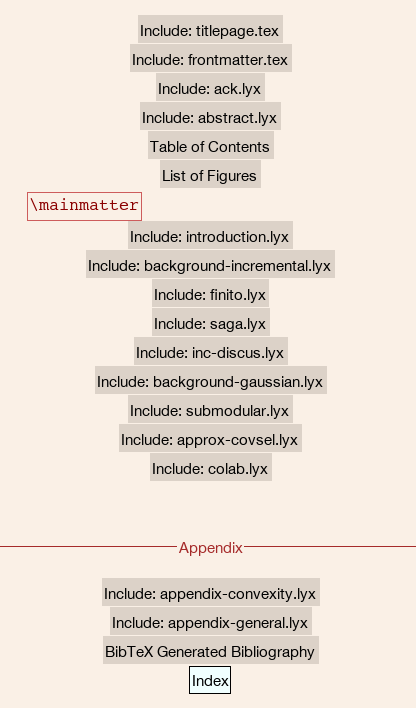### Setting up the main document

Create a new document, and go to Document->Settings->LaTeX Preamble, put in the preamble files we created above:
\input{general-preamble}
\input{main-preamble}

Each of the child documents is included via Insert->File->LyX Document ... The “include type” needs to be set to “include” for it to work correctly. The \mainmatter command signifies the switch over from page numbering using Roman numerals (for the introductory material) to Arabic numerals (for the thesis proper). It is inserted using the “TeX Code” insertion (Ctrl-L), which just directly inserts LaTeX commands into the document.
The Append is started with Document->Start Appendix Here, which should probably be in the insert menu of LyX instead. The bibliography is created with Insert->List/TOC->BibTex bibliography.., which lets you select a BibTex .bib file to use for your citations. LyX supports using multiple different bib files, which would appear useful if your combining multiple papers you have written into a thesis. A word of caution: if there are repeated entries between the bib files you will run into various hard to debug problems in LyX, especially if the entries only differ in capitalisation. I would suggest taking all the bib files and merging them using a command line tool into one file. I used the command:
bibtool -s -d *.bib > all.bib

LyX obeys most of the styling information specified by the preamble we created above. However, there are a few things that it overrides. Follow steps 3-7 from the next section to fix these up.

### Setting up the child documents

In my case I put each child document in it’s own folder. This is not necessary, but it seemed like a good idea to keep the child documents in a folder together with any figures used by that document. I will walk through the creation of a single child document here. I created all the chapters initially by just copying the first one I created, but you could do it in a more organised way using LyX templates. The steps for creating the chapter are:
1. Create a new empty LyX document and save it to a folder with the chapter within your thesis directory.
2. Add the document to your main document using the include procedure described above.
3. Open up the settings of the chapter document, and set the document class to Book, and in the master document field, point it at your main.lyx document4. On the font section, change the default family base font size to what ever your university requires. This was different than the default for me.
5. Tick the Two-sided document check-box under Page Layout. This sets it so that the margin is wider on the outside-edge of each page, so it looks right when bound as a book.
6. On the Language section, change it to English (Australian) or English (UK) if your not in the ’ol US of A.
7. Your university almost certain specifies a particular citation style. Specify that in the Bibliography section. My university (ANU) recommends a Author-year style:8. In the LaTeX Preamble, add the command \input{../general-preamble}, which just points to the previously created preamble file. The main-preamble file is not used by the child documents.
9. If your using sub-folders for each chapter, place a copy of the thesis.sty and (for ANU) the anuthesis.sty files in the subfolder as well.
The above setup will mean that you can view each chapter separately in LyX using the eye button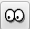in the toolbar. The chapter can be viewed within the whole-thesis PDF using the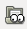button. When viewing separately, the bibliography references will display as question marks (?), whereas they will be displayed correctly in the whole-thesis PDF. There are some suggested work-arounds for this issue on on the LyX Wiki, but I couldn’t get them to work.

## Other productivity enhancements

There is a few additional setup steps you should go through in LyX if you haven’t already. These are not required, but they will generally increase your productivity.
• Setup Forward and Reverse Search
• Setup groups of chapters as LyX branches to make sending subsets of your thesis easier
• Setup math macros
• Add keyboard shortcuts for citations and cross-references.

# Math Macros: The best feature of Lyx you’re not using

$\newcommand{\lyxlock}{}$
LyX is a brilliant tool for writing in the mathematical sciences. It provides a WYSIWYG style editor on top of LaTeX with just the right amount of abstraction for getting-stuff-done. With the proper setup, typing math into LyX is easier, faster and less error prone then using LaTeX directly, and it has virtually no down sides.
Part of the setup is the use of use of LyX math macros. Math macros allow you to define new commands that you can use within LyX math mode. When writing pure LaTeX, most people include a set of standard macros in the header of the LaTeX documents. For example, a common one is a shortcut for argmin:
\newcommand{\argmin}{\operatornamewithlimits{argmin}}

LyX math macros allow you to do the same thing within LyX.
To create the equivalent macro in LyX, create a new math macroThis will insert the following into your document: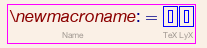This won’t appear when you render out your document, so you can put it anywhere you like. To create the macro, just fill out the 3 parts. The first part is what you will type when using the macro, the second part is the LaTeX that will be output, and the third part controls what it looks like on screen within LyX. For most macros the TeX and LyX sections should be the same, for the argmin example we want the TeX code to produce a “mathop”, which LyX can’t natively display. So we put a \TeXtrm{argmin} in the LyX part. The filled in macro is thus: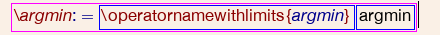Note that for this to work you have to have amsmath package imported. To set that up, go Document -> Settings..., then select the radio buttons under the math options section: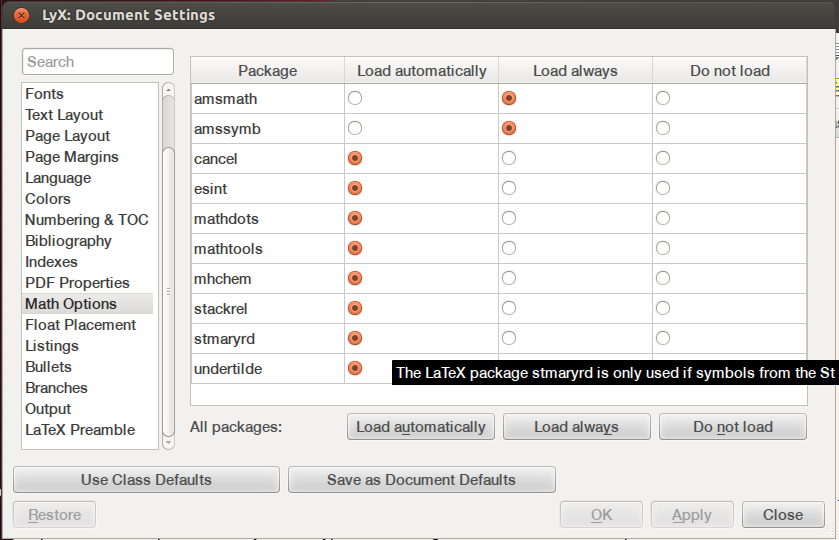To use the macro, just type \argmin within a LyX math box, then press space. It should insert the macro seemlessly. Within LyX the subscripts will look like:
but when you render it out to a pdf it will be typeset correctly as:

## A few useful macros

I have the following macros setup in a lyx-math-macros.lyx file: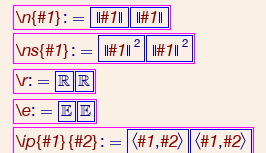These macros illustrate the parameters feature as well. To add parameters to a macro, use the macro bar that appears at the bottom of the screen when editing the macro.
I have a lyx template setup that I use for new documents. It includes a LyX sub-document include statement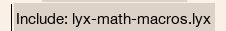which gives access to the macros.

## How it’s implemented

If you convert your LyX document to a pdfLaTeX document, you’ll see the following command is generated in the preamble
\global\long\def\argmin{\operatornamewithlimits{argmin}}

This uses lower level LaTeX commands then \newcommand, but it has a similar effect . At the call site you’ll see something like:
{argmin}_{x\in \mathbb{R}}

Other then the extra {} brackets, this is as simple as you could want.